#1. 云栖社区>
2. 翻译小组>
3. 博客>
4. 正文

## 手把手教你监督学习（附python实战代码）

【方向】 2018-05-17 19:59:51 浏览10345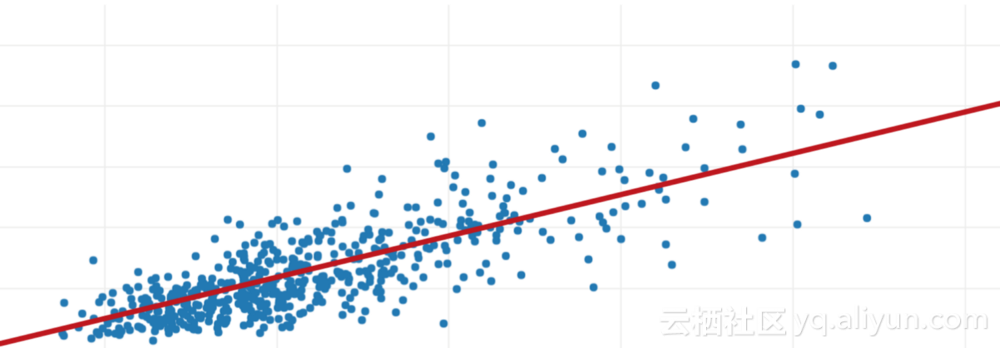X是INPUT（他准备的小时数）；

Y是输出（标记在考试中得分的学生）；

C将是随机错误。

### 分类

#### 分类的最佳方法

1.K-最近邻；

2.决策树；

3.朴素贝叶斯；

4.支持向量机；

``````pip install pandas
pip install matplotlib
pip install scikit-learn``` ```

``````from sklearn import datasets
import pandas as pd
import matplotlib.pyplot as plt
# Loading IRIS dataset from scikit-learn object into iris variable.
# Prints the type/type object of iris
print(type(iris))
# <class 'sklearn.datasets.base.Bunch'>
# prints the dictionary keys of iris data
print(iris.keys())
# prints the type/type object of given attributes
print(type(iris.data), type(iris.target))
# prints the no of rows and columns in the dataset
print(iris.data.shape)
# prints the target set of the data
print(iris.target_names)
# Load iris training dataset
X = iris.data
# Load iris target set
Y = iris.target
# Convert datasets' type into dataframe
df = pd.DataFrame(X, columns=iris.feature_names)
# Print the first five tuples of dataframe.

``````<class ‘sklearn.datasets.base.Bunch’>
dict_keys([‘data’, ‘target’, ‘target_names’, ‘DESCR’, ‘feature_names’])]
<class ‘numpy.ndarray’> <class ‘numpy.ndarray’>
(150, 4)
[‘setosa’ ‘versicolor’ ‘virginica’]
sepal length (cm) sepal width (cm) petal length (cm) petal width  (cm)
0   5.1   3.5   1.4  0.2
1   4.9   3.0   1.4  0.2
2   4.7   3.2   1.3  0.2
3   4.6   3.1   1.5  0.2
4   5.0   3.6   1.4  0.2``````

### Scikit-learn中的K-最近邻居

K-最近邻分类器就是一个懒惰的学习者。

KNN基于类比学习，即将给定的测试元组与类似的训练元组进行比较。训练元组由n个属性描述，每个元组代表一个n维空间中的一个点。这样，所有训练元组都存储在n维模式空间中。当给定未知元组时，k-最近邻分类器在模式空间中搜索最接近未知元组的k个训练元组。这k个训练元组是k未知元组的k个“最近邻居”。

``````from sklearn import datasets
from sklearn.neighbors import KNeighborsClassifier

# Load iris dataset from sklearn

# Declare an of the KNN classifier class with the value with neighbors.
knn = KNeighborsClassifier(n_neighbors=6)

# Fit the model with training data and target values
knn.fit(iris['data'], iris['target'])

# Provide data whose class labels are to be predicted
X = [
[5.9, 1.0, 5.1, 1.8],
[3.4, 2.0, 1.1, 4.8],
]

# Prints the data provided
print(X)

# Store predicted class labels of X
prediction = knn.predict(X)

# Prints the predicted class labels of X
print(prediction)``````

[1 1]

1对应Virginic

2对应Setosa

#### KNN直观的IRIS数据集分类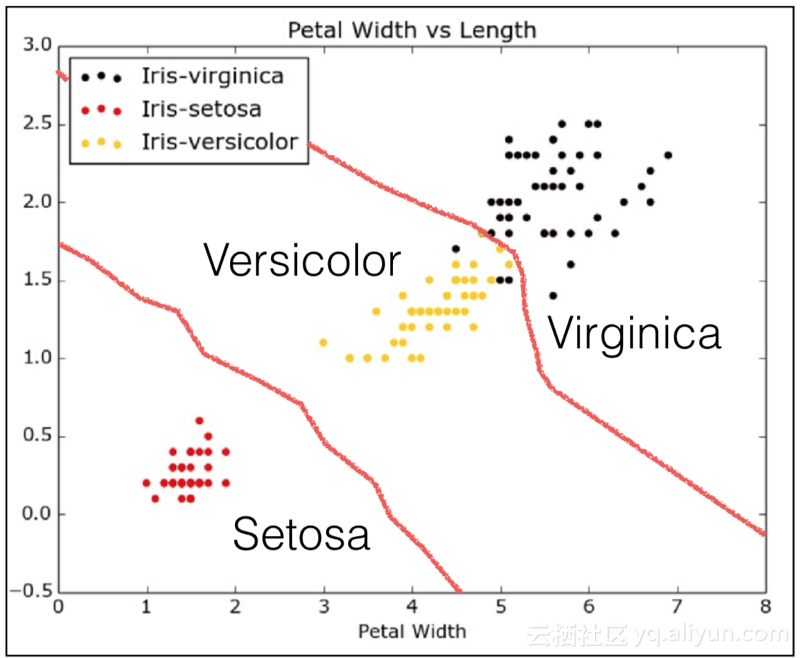### 回归

·线性回归

·Logistic回归

·多项式回归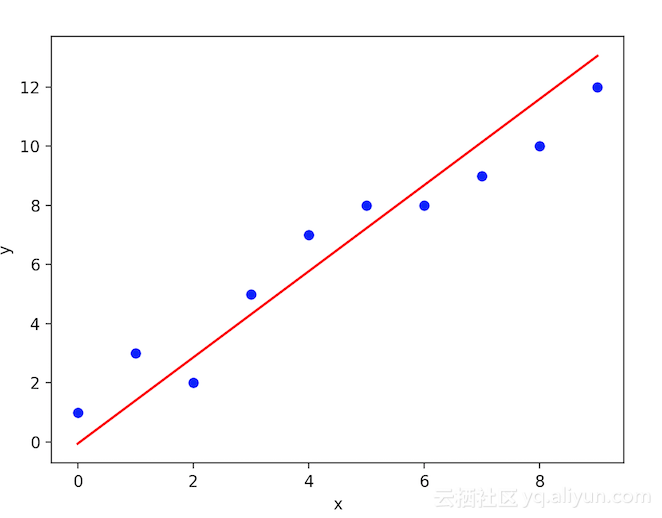Logistic Regression是一种算法，用于响应变量是分类的地方。Logistic回归的想法是找出特征与特定结果的概率之间的关系。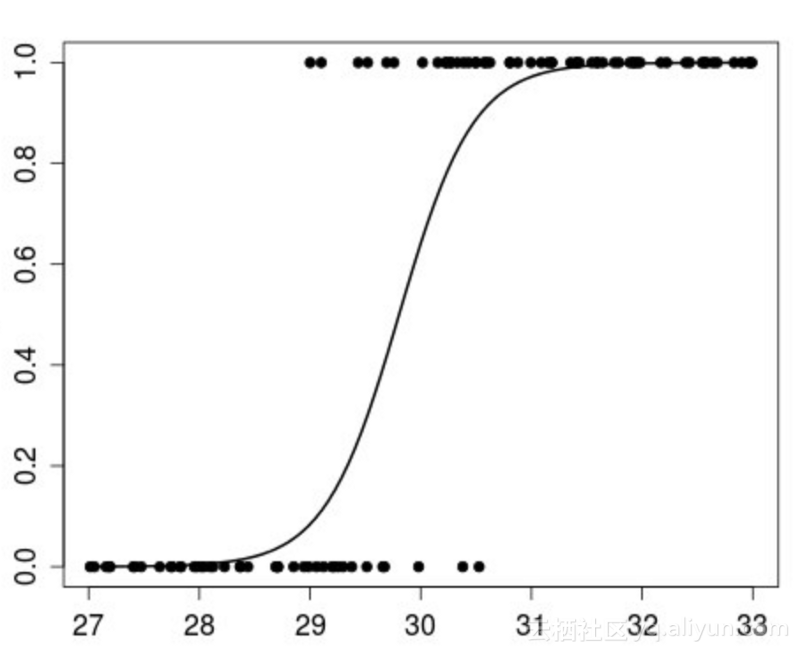``````from sklearn import datasets, linear_model
import matplotlib.pyplot as plt
import numpy as np
# Load the diabetes dataset
# Use only one feature for training
diabetes_X = diabetes.data[:, np.newaxis, 2]
# Split the data into training/testing sets
diabetes_X_train = diabetes_X[:-20]
diabetes_X_test = diabetes_X[-20:]
# Split the targets into training/testing sets
diabetes_y_train = diabetes.target[:-20]
diabetes_y_test = diabetes.target[-20:]
# Create linear regression object
regr = linear_model.LinearRegression()
# Train the model using the training sets
regr.fit(diabetes_X_train, diabetes_y_train)
# Input data
print('Input Values')
print(diabetes_X_test)
# Make predictions using the testing set
diabetes_y_pred = regr.predict(diabetes_X_test)
# Predicted Data
print("Predicted Output Values")
print(diabetes_y_pred)
# Plot outputs
plt.scatter(diabetes_X_test, diabetes_y_test, color='black')
plt.plot(diabetes_X_test, diabetes_y_pred, color='red', linewidth=1)
plt.show()``````

``````Input Values
[
[ 0.07786339]  [-0.03961813]  [ 0.01103904]  [-0.04069594]    [-0.03422907]  [ 0.00564998]  [ 0.08864151]  [-0.03315126] [-0.05686312]  [-0.03099563]  [ 0.05522933]  [-0.06009656]
[ 0.00133873]  [-0.02345095]  [-0.07410811]  [ 0.01966154][-0.01590626]  [-0.01590626]  [ 0.03906215]  [-0.0730303 ]
]
Predicted Output Values
[
225.9732401   115.74763374  163.27610621  114.73638965   120.80385422  158.21988574  236.08568105  121.81509832
99.56772822   123.83758651  204.73711411   96.53399594
154.17490936  130.91629517   83.3878227   171.36605897
137.99500384  137.99500384  189.56845268   84.3990668
]``````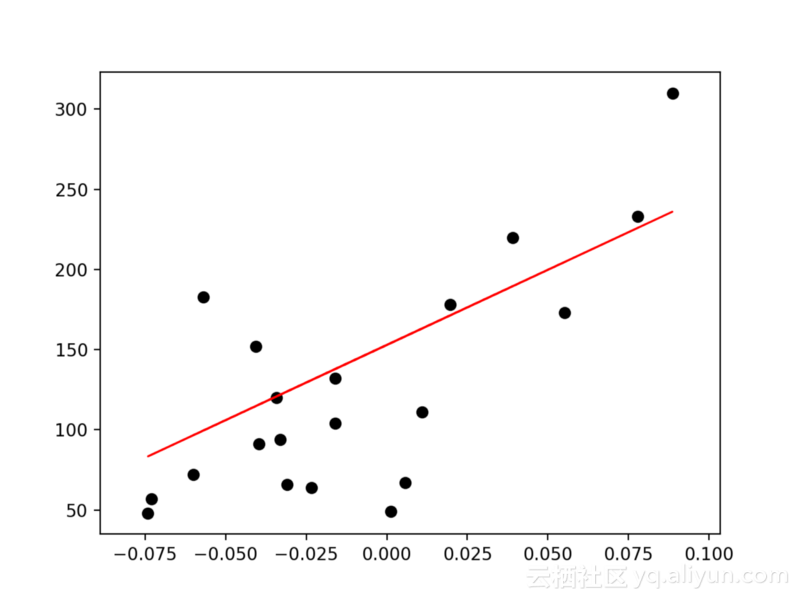（糖尿病_X_测试，糖尿病_y_pred）预测之间的图将在线方程上连续。